# KVPY-SA 2016 Chemistry Paper with Solutions

KVPY-SA 2016 Chemistry question paper along with solutions for all the asked questions are available on this page. The solution to each of the questions is given in detail to cater to students' need for understanding the concept more clearly. BYJU'S subject matter experts have solved the questions in a simple manner which will help students to grasp the answer easily. Meanwhile, if we look at the general trend of Chemistry paper, students will need to have an in-depth understanding of all the related concepts. This is where solving question papers can help. They will not only get a clear idea of the topic but it will help them to conduct meaningful revisions before the main exam
Students can also download KVPY SA Chemistry 2016 question paper and solutions in PDF format and practise well before the exams.

### KVPY SA 2016 - Chemistry

Question 1: One mole of one of the sodium salts listed below, having carbon content close to 14.3% produces 1 mole of carbon dioxide upon heating (atomic mass Na = 23, H = 1, C = 12, O = 16). The salt is

1. a) C2H5COONa
2. b) NaHCO3
3. c) HCOONa
4. d) CH3COONa

Solution:

$2NaHCO_3 \overset{\Delta}{\rightarrow} Na_2CO_3 + H_2O + CO_2$

Molecular weight of NaHCO3 = 23+1+12+48 = 84 g mol–1

Molecular weight of carbon = 12 g mol–1

% carbon content in NaHCO3 = (12/84) x 100 = 14.28%

The C content in other compound is not 14.3%

Question 2: Among formic acid, acetic acid, propanoic acid and phenol, the strongest acid in water is

1. a) formic acid
2. b) acetic acid
3. c) propanoic acid
4. d) phenol

Solution:

Formic acid is strongest acid

→ Carboxylic acids are more acidic than phenol due to equivalent resonating structure.

E.g.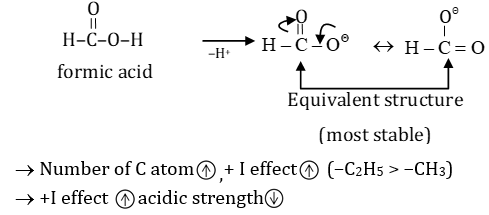→ Carboxylic acid is more acidic than phenol and alcohol because conjugate base of a carboxylic acid is stabilized by two equivalent resonance structures in which the negative charge is effectively delocalized between two more electronegative oxygen atoms.

→ Hence, the overall order of acidic strength will be –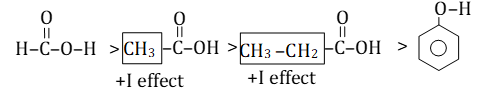Question 3: According to Graham's Law, the rate of diffusion of CO, O2, N2 and CO2 follows the order:

1. a) CO = N2 > O2 > CO2
2. b) CO = N2 > CO2 > O2
3. c) O2 > CO = N2 > CO2
4. d) CO2 > O2 >CO = N2

Solution:

Rate of diffusion ∝ 1/√ m, m → molecular weight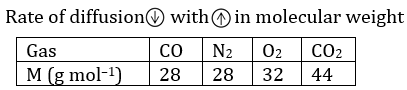Hence, the order of molecular mass → CO2 > O2 > CO=NO

Order of diffusion CO= N2 > O2 > CO2

Question 4: The major product formed when 2-butene is reacted with O3 followed by treatment with Zn/H2O is

1. a) CH3COOH
2. b) CH3CHO
3. c) CH3CH2OH
4. d) CH2 = CH2

Solution:

Reaction of 2-butene with O2 follow as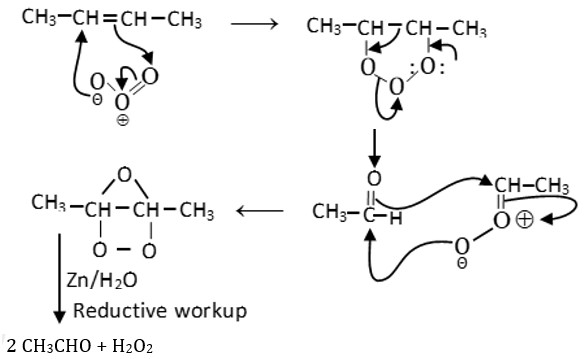Question 5: The IUPAC name for the following compound is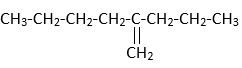1. a) 2-propylhex-1-ene
2. b) 2-butylpent-1-ene
3. c) 2-propyl-2- butylethene
4. d) Propyl-1-butylethene

Solution:

Solution: (2 propyl hex -1-ene)

Question 6: The major products obtained in the reaction of oxalic acid with conc. H2SO4 upon heating are

1. a) CO, CO2, H2O
2. b) CO, SO2, H2O
3. c) H2S, CO, H2O
4. d) HCOOH, H2S, CO

Solution:

Reaction of oxalic acid with conc. H2SO4 upon heating follows as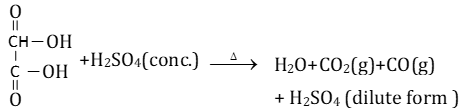Question 7: LiOH reacts with CO2 to form Li2CO3 (atomic mass of Li=7). The amount of CO2 (in g) consumed by 1g of LiOH is closest to

1. a) 0.916
2. b) 1.832
3. c) 0.544
4. d) 1.088

Solution:

Reaction of LiOH with CO2 follows as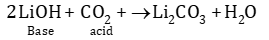Hence, the moles of LiOH = moles of CO2 × 2

Moles of LiOH = (weight of LiOH)/(molecular wt) = 1/24 = 0.417 moles

Hence, the moles of CO2= (1/2) x moles of LiOH = 0.0208 moles

Weight of CO2 = moles x molecular weight = 0.0208 × 44 = 0.916 g

Therefore, the correct option is (A).

Question 8: The oxidation number of sulphur is +4 in

1. a) H2S
2. b) CS2
3. c) Na2SO4
4. d) Na2SO3

Solution:

Let, the oxidation number of S = X

(i) H2S → x+2 x(+1) = 0 ⇒ x = –2

(ii) CS2 → 2x + 1 x (+4) = 0 ⇒ x =-2

(iii) Na2SO4 → 2 x(+1)+x+(-2)x4 = 0 ⇒ x = +6

(iv) Na2SO3 → 2 x (+1)+x +(-2)x3 = 0 ⇒ x = +4

[*Using oxidation number of H,C, Na and O]

Question 9: Al2O3 reacts with

1. a) only water
2. b) only acids
3. c) only alkalis
4. d) both acids and alkalis

Solution:

Hint: See the definition and key point of amphoteric oxide.

Al2O3 is an amphoteric oxide hence, it reacts with both acid and base as given below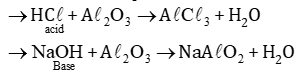Option (D) is correct.

Question 10: The major product formed in the oxidation of acetylene by alkaline KMnO4 is

1. a) Ethanol
2. b) acetic acid
3. c) formic acid
4. d) oxalic acid

Solution: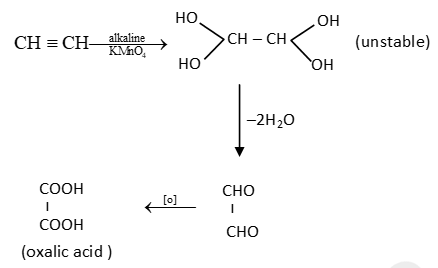Question 11: In a closed vessel, an ideal gas at 1 atm is heated from 27°C to 327°C. The final pressure of the gas will approximately be

1. a) 3 atm
2. b) 0.5 atm
3. c) 2 atm
4. d) 12 atm

Solution:

According to the Gay-Lussac’s law, at constant volume, pressure of gas is directly proportional to the absolute temperature of gas (v,n,R -> constant).

P ∝ T or P = KT

P1 = T1 K, P2 = T2 K

P1/P2 = T1/T2

Where, P1 is initial pressure

P2 is final pressure

T1 is initial temperature

T2 is final temperature

1/P2 = 300/600

P2 = 2 atm

Question 12: Among the element Li, N, C and Be, one with the largest atomic radius is

1. a) Li
2. b) N
3. c) C
4. d) Be

Solution: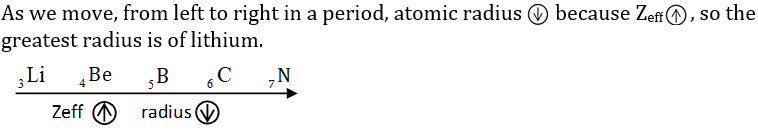Question 13: A redox reaction among the following is

1. a) CdCl2 + 2KOH → Cd (OH)2 + 2KCl
2. b) BaCl2 + K2SO4 → BaSO4 + 2KCl
3. c) CaCO3 → CaO + CO2
4. d) 2Ca + O2 → 2CaO

Solution:

Redox Reaction ->

Chemical reaction in which oxidation and reduction process takes place simultaneously known as redox reaction.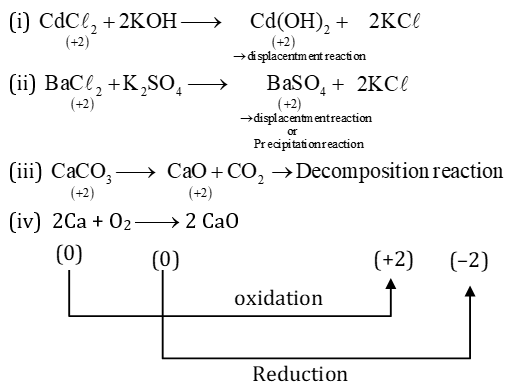Hence, it is a redox reaction.

Question 14: The electronic configuration which obeys Hund's rule for the ground state of carbon atom is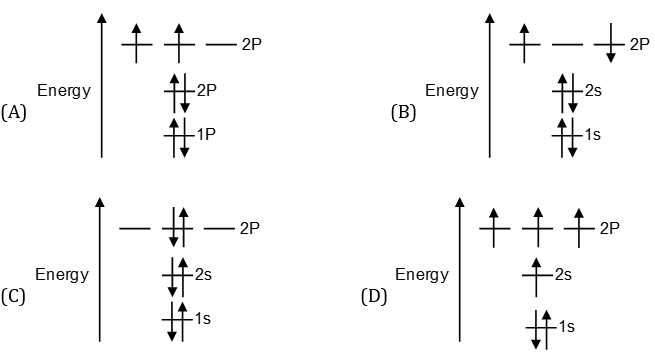Solution:

Hund’s Rules ->

According to Hund’s rule, in the ground state of an atom/molecules, the electronic configuration with lowest energy, is the one with the highest value of spin multiplicity. This implies that low energy orbital’s are first completely filled and in case of degenerate orbital’s (two or more orbital’s of equal energy), each orbital occupy single electron with same spin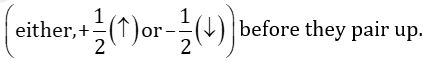Energy of orbital’s 1S < 2S < 2P

If we see all the options, only C follows the Hund’s law according above definition.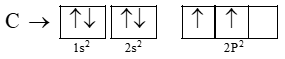Question 15: The graph that depicts Einstein photoelectric effect for a monochromatic source of frequency above the threshold frequency is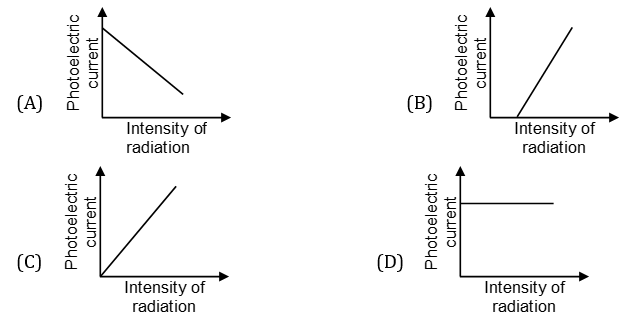Solution:

Photoelectric effect is the phenomenon of ejection of electron from the surface of a material upon irradiation with light. The ejected electron thus, gives the photo electric current. These electrons can be ejected only by supplying a minimum amount of energy called threshold energy (frequency). Intensity of a monochromatic light is proportional to the number of photons with particular frequency. Since, each photon can abstract one electron at a time therefore, if the photons, the number of photoelectrons in the same proportion. Thus, suggests that above threshold frequency the photoelectric current and light intensity will linearly.

Question 16: In the following reactions, X, Y and Z are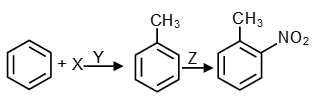1. a) X = CH3Cl; Y = anhydrous AlCl3; Z = HNO3 + H2SO4
2. b) X = CH3COCl; Y = anhydrous AlCl3; Z = HNO3 + H2SO4
3. c) X = CH3Cl; Y = conc. H2SO4; Z = HNO3 + H2SO4
4. d) X = CH3Cl; Y = dil. H2SO4; Z = HNO3

Solution: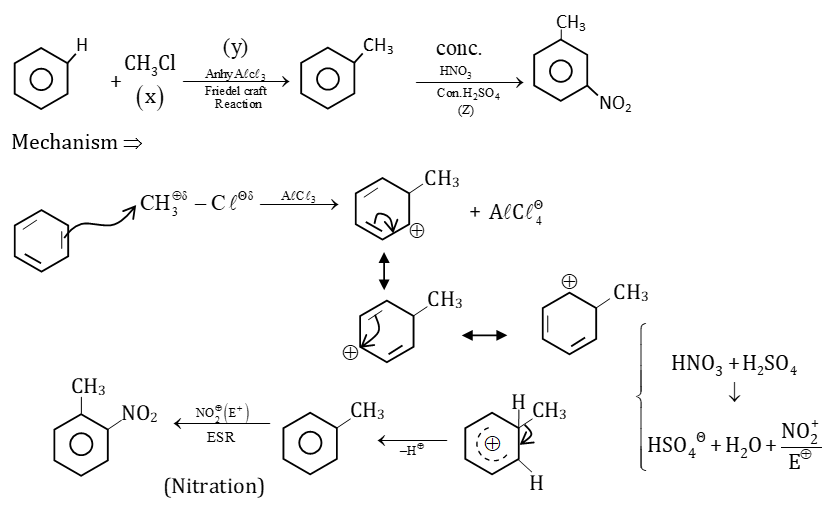Question 17: 2,3-dibromobutane can be converted to 2-butyne in two-step reaction using

1. a) (i) HCl and (ii) NaH
2. b) (i) alcoholic KOH and (ii) NaNH2
3. c) (i) Na and (ii) NaOH
4. d) (i) Br2 and (ii) NaH

Solution:

Reaction mechanism of conversion of 2,3 dibromo butane to 2 butyne follow as–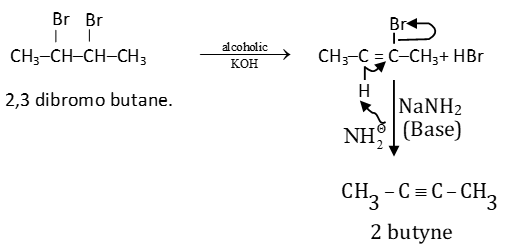Question 18: Given

NO(g) + O3 (g) → NO2 (g) + O2 (g) and Δ H = –198.9 kJ/mol

O3(g) → 3/2 O2 (g) and ΔH = –142.3 kJ/mol

O2(g) → 2O(g) and ΔH = +495.0 kJ/mol

The enthalpy change (Δ H) for the following reaction is

NO (g) + O (g) → NO2 (g)

1. a) –304.1 kJ/mol
2. b) +304.1 kJ/mol
3. c) –403.1 kJ/mol
4. d) +403.1 kJ/mol

Solution: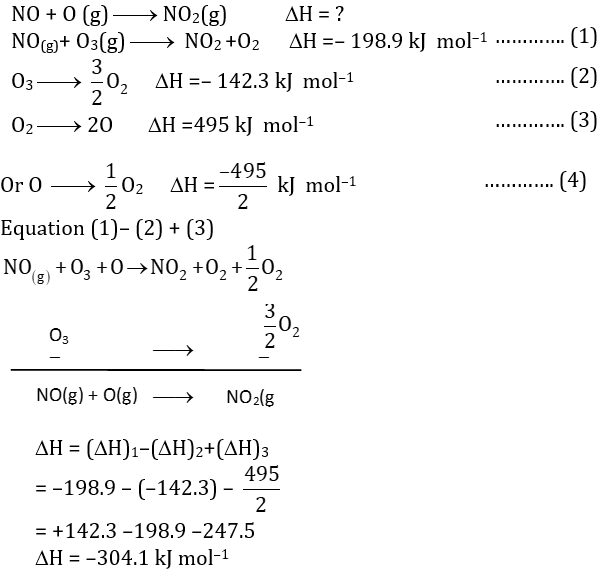Note: Enthalpy is a state function hence, it depends only on initial and final state, and not on path followed by the function.

Therefore, the correct answer is (A).

Question 19: A 1.85 g sample of an arsenic-containing pesticide was chemically converted to AsO43– (atomic mass of As = 74.9) and titrated with Pb2+ to form Pb3 (AsO4)2. If 20 mL of 0.1 M Pb2+ is required to reach the equivalence point, the mass percentage of arsenic in the pesticide sample is closest to

1. a) 8.1
2. b) 2.3
3. c) 5.4
4. d) 3.6

Solution:

Reaction of Pb+2 with ASO3-4 follows as

3Pb+2 + 2 ASO3-4 -> Pb3 (ASO4)2

From above reaction,

Moles of Pb+2 = (3/2) moles of ASO3-4

Moles of ASO3-4 = (2/3) moles of Pb+2

Morality of Pb+2 = (moles x1000)/v

Moles of Pb+2 = (0.1 x 20)/1000 = 2 x 10-3 moles

Hence, moles of ASO3-4 = (2/3) x 2 x 10-3 = (4/3) x 10-3 moles

Molecular weight of As = 74.9 g mol–1

Because, moles = wt/mw, so wt of As = moles x mw = 74.9 x 4/3 x 10-3

Weight of As = 0.09986g

Therefore, Percentage of As in sample = (0.09986/1.85) x 100 = 5.4%

Question 20: When treated with conc. HCl, MnO2 yields gas (X) which further reacts with Ca(OH2) to generate a white solid (Y). The solid (Y) reacts with dil. HCl to produce the same gas X. the solid Y is

1. a) CaO
2. b) CaCl2
3. c) Ca(OCl)Cl
4. d) CaCO3

Solution:

Following steps are involved in the reaction -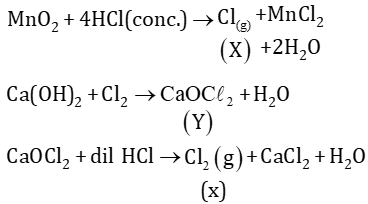Hence, here solid Y = Ca(OCl) Cl or CaOCl2

Therefore, the correct answer is (C).

### KVPY-SA 2016 Chemistry Paper with Solutions